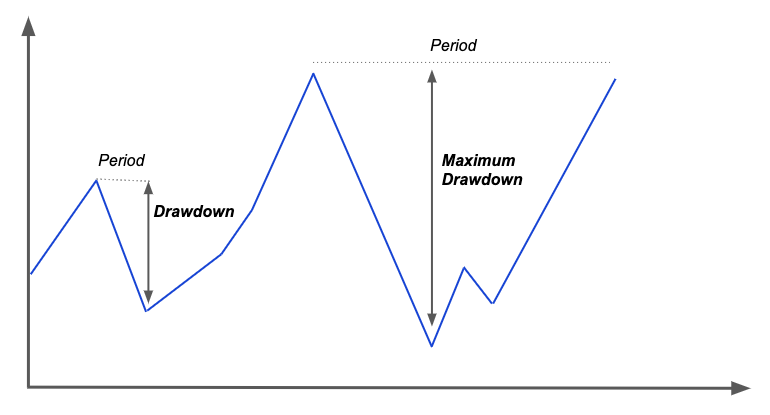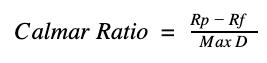# Calmar Ratio: Definition, Calculation, and Importance

Calmar ratio is a popular risk-adjusted measure used by investors in their investment selection process. The Calmar ratio is calculated by dividing the compounded annual rate of return for period and dividing it by the maximum drawdown for the same period. The calculations are done using absolute values. Calmar ratios are generally calculated using 36 months of data. It is calculated on a monthly basis. The lower the Calmar Ratio, the worse the performance of the investment; the higher the Calmar Ratio, the better the performance. Among traders, a Calmar ratio of 1plus is considered good, 3 plus is excellent and 5 plus is awesome.

For Calmar ratio, the unit of risk is the maximum drawdown.Drawdowns consist of peak-to-trough declines during a specific period of investment.  The period between the peak level and the trough is called the “length” of the drawdown. Maximum drawdown is defined as the maximum loss from a peak-to-trough during an investment period.### FormulaWhere:

• Rp is the portfolio returns
• Rf if the risk-free rate
• Max D is the maximum drawdown for the period

Calmar ratio has the effect of smoothing out the overachievements and underachievements in a portfolio. Essentially, the Calmar ratio normalizes the returns of a portfolio by its tail risk. Since it does a good job of smoothing performance results, it encourages long-term investment outlook. It can also be used as a warning signal. If the Calmar ratio is on a downtrend, it is important for investors to review their portfolio to find whether the decrease is because of increased volatility, decreased returns or some other factors.

Calmar ratio is also an important ratio calculated while backtesting trading strategies. Investors will generally use Calmar ratio in conjunction with Sharpe Ratio and Sterling Ratio. Unlike the Sharpe Ratio, the Calmar Ratio cannot be scaled to different time horizons, so portfolios that use the Calmar Ratio should have the same backtesting period.